Courses

# Instruction Pipelining Computer Science Engineering (CSE) Notes | EduRev

## GATE : Instruction Pipelining Computer Science Engineering (CSE) Notes | EduRev

The document Instruction Pipelining Computer Science Engineering (CSE) Notes | EduRev is a part of the GATE Course GATE Computer Science Engineering(CSE) 2022 Mock Test Series.
All you need of GATE at this link: GATE

## Pipelining | (Execution, Stages and Throughput)

To improve the performance of a CPU we have two options:
1) Improve the hardware by introducing faster circuits.
2) Arrange the hardware such that more than one operation can be performed at the same time.

Since, there is a limit on the speed of hardware and the cost of faster circuits is quite high, we have to adopt the 2nd option.

Pipelining : Pipelining is a process of arrangement of hardware elements of the CPU such that its overall performance is increased. Simultaneous execution of more than one instruction takes place in a pipelined processor.

Let us see a real life example that works on the concept of pipelined operation. Consider a water bottle packaging plant. Let there be 3 stages that a bottle should pass through, Inserting the bottle, Filling water in the bottle, and Sealing the bottle. Let us consider these stages as stage 1, stage 2 and stage 3 respectively. Let each stage take 1 minute to complete its operation.
Now, in a non pipelined operation, a bottle is first inserted in the plant, after 1 minute it is moved to stage 2 where water is filled. Now, in stage 1 nothing is happening. Similarly, when the bottle moves to stage 3, both stage 1 and stage 2 are idle. But in pipelined operation, when the bottle is in stage 2, another bottle can be loaded at stage 1. Similarly, when the bottle is in stage 3, there can be one bottle each in stage 1 and stage 2. So, after each minute, we get a new bottle at the end of stage 3. Hence, the average time taken to manufacture 1 bottle is :

Without pipelining = 1 min + 1 min + 1 min = 3 minutes
With pipelining = 1 minute

Thus, pipelined operation increases the efficiency of a system.

Design of a basic pipeline

• In a pipelined processor, a pipeline has two ends, the input end and the output end. Between these ends, there are multiple stages/segments such that output of one stage is connected to input of next stage and each stage performs a specific operation.
• Interface registers are used to hold the intermediate output between two stages. These interface registers are also called latch or buffer.
• All the stages in the pipeline along with the interface registers are controlled by a common clock.

Execution in a pipelined processor

Execution sequence of instructions in a pipelined processor can be visualized using a space-time diagram. For example, consider a processor having 4 stages and let there be 2 instructions to be executed. We can visualize the execution sequence through the following space-time diagrams: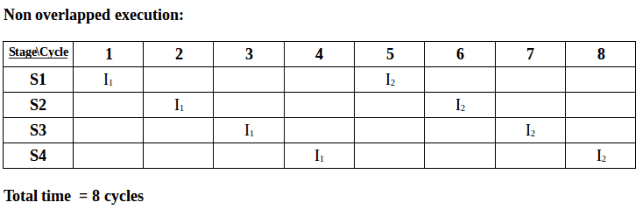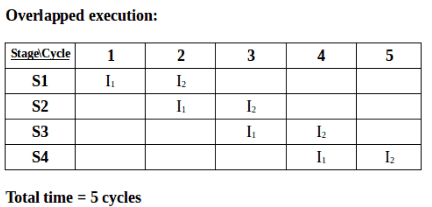Pipeline Stages

RISC processor has 5 stage instruction pipeline to execute all the instructions in the RISC instruction set. Following are the 5 stages of RISC pipeline with their respective operations:

• Stage 1 (Instruction Fetch)
In this stage the CPU reads instructions from the address in the memory whose value is present in the program counter.
• Stage 2 (Instruction Decode)
In this stage, instruction is decoded and the register file is accessed to get the values from the registers used in the instruction.
• Stage 3 (Instruction Execute)
In this stage, ALU operations are performed.
• Stage 4 (Memory Access)
In this stage, memory operands are read and written from/to the memory that is present in the instruction.
• Stage 5 (Write Back)
In this stage, computed/fetched value is written back to the register present in the instruction.

Performance of a pipelined processor

Consider a ‘k’ segment pipeline with clock cycle time as ‘Tp’. Let there be ‘n’ tasks to be completed in the pipelined processor. Now, the first instruction is going to take ‘k’ cycles to come out of the pipeline but the other ‘n – 1′ instructions will take only ‘1’ cycle each, i.e, a total of ‘n – 1′ cycles. So, time taken to execute ‘n’ instructions in a pipelined processor:

ETpipeline = k + n – 1 cycles
= (k + n – 1) Tp

In the same case, for a non-pipelined processor, execution time of ‘n’ instructions will be:

ETnon-pipeline = n * k * Tp

So, speedup (S) of the pipelined processor over non-pipelined processor, when ‘n’ tasks are executed on the same processor is:

S = Performance of pipelined processor / Performance of Non-pipelined processor

As the performance of a processor is inversely proportional to the execution time, we have,

S = ETnon-pipeline / ETpipeline
=> S =  [n * k * Tp] / [(k + n – 1) * Tp]
S = [n * k] / [k + n – 1]

When the number of tasks ‘n’ are significantly larger than k, that is, n >> k

S ≈ n * k / n
S ≈ k

where ‘k’ are the number of stages in the pipeline.

Also, Efficiency = Given speed up / Max speed up = S / Smax
We know that, Smax = k

So, Efficiency = S / k

Throughput = Number of instructions / Total time to complete the instructions

So, Throughput = n / (k + n – 1) * Tp

Note: The cycles per instruction (CPI) value of an ideal pipelined processor is 1

## Pipelining | (Dependencies and Data Hazard)

Dependencies in a pipelined processor

There are mainly three types of dependencies possible in a pipelined processor. These are :
1) Structural Dependency
2) Control Dependency
3) Data Dependency

These dependencies may introduce stalls in the pipeline.

Stall : A stall is a cycle in the pipeline without new input.

Structural dependency

This dependency arises due to the resource conflict in the pipeline. A resource conflict is a situation when more than one instruction tries to access the same resource in the same cycle. A resource can be a register, memory, or ALU.

Example: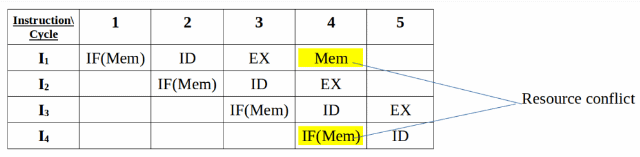In the above scenario, in cycle 4, instructions I1 and I4 are trying to access same resource (Memory) which introduces a resource conflict.
To avoid this problem, we have to keep the instruction on wait until the required resource (memory in our case) becomes available. This wait will introduce stalls in the pipeline as shown below: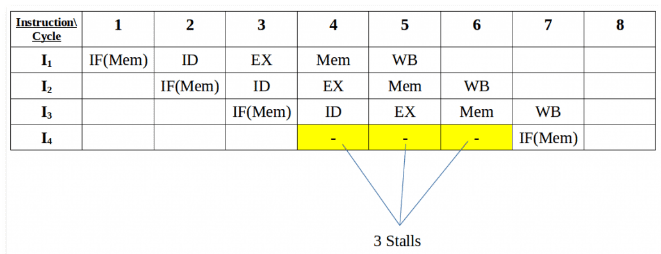Solution for structural dependency

To minimize structural dependency stalls in the pipeline, we use a hardware mechanism called Renaming.
Renaming : According to renaming, we divide the memory into two independent modules used to store the instruction and data separately called Code memory(CM) and Data memory(DM) respectively. CM will contain all the instructions and DM will contain all the operands that are required for the instructions.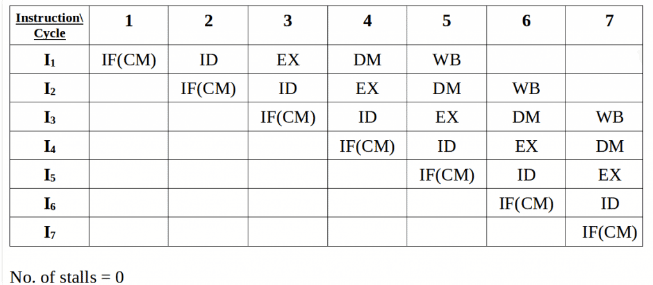Control Dependency (Branch Hazards)

This type of dependency occurs during the transfer of control instructions such as BRANCH, CALL, JMP, etc. On many instruction architectures, the processor will not know the target address of these instructions when it needs to insert the new instruction into the pipeline. Due to this, unwanted instructions are fed to the pipeline.

Consider the following sequence of instructions in the program:
100: I1
101: I2 (JMP 250)
102: I3
250: BI1

Expected output: I1 -> I2 -> BI1

NOTE: Generally, the target address of the JMP instruction is known after ID stage only.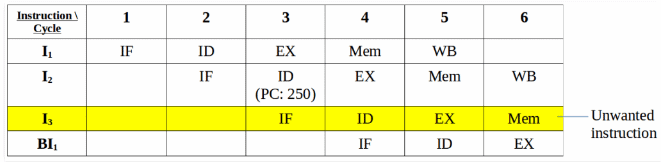Output Sequence: I1 -> I2 -> I3 -> BI1

So, the output sequence is not equal to the expected output, that means the pipeline is not implemented correctly.

To correct the above problem we need to stop the Instruction fetch until we get target address of branch instruction. This can be implemented by introducing delay slot until we get the target address.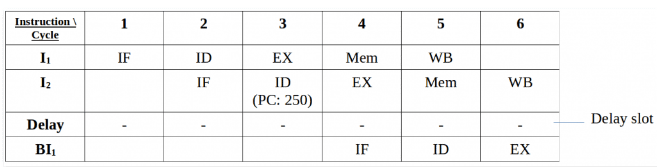Output Sequence: I1 -> I2 -> Delay (Stall) -> BI1

As the delay slot performs no operation, this output sequence is equal to the expected output sequence. But this slot introduces stall in the pipeline.

Branch penalty : The number of stalls introduced during the branch operations in the pipelined processor is known as branch penalty.

NOTE : As we see that the target address is available after the ID stage, so the number of stalls introduced in the pipeline is 1. Suppose, the branch target address would have been present after the ALU stage, there would have been 2 stalls. Generally, if the target address is present after the kth stage, then there will be (k – 1) stalls in the pipeline.

Total number of stalls introduced in the pipeline due to branch instructions = Branch frequency * Branch Penalty

Data Dependency (Data Hazard)

Let us consider an ADD instruction S, such that
S : ADD R1, R2, R3
Addresses written by S = O(S) = {R1}

Now, we say that instruction S2 depends in instruction S1, when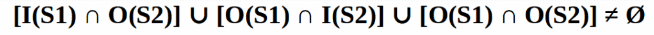This condition is called Bernstein condition.

Three cases exist:

• Flow (data) dependence: O(S1) ∩ I (S2), S1 → S2 and S1 writes after something read by S2
• Anti-dependence: I(S1) ∩ O(S2), S1 → S2 and S1 reads something before S2 overwrites it
• Output dependence: O(S1) ∩ O(S2), S1 → S2 and both write the same memory location.

Example: Let there be two instructions I1 and I2 such that:
I1 : ADD R1, R2, R3
I2 : SUB R4, R1, R2

When the above instructions are executed in a pipelined processor, then data dependency condition will occur, which means that I2 tries to read the data before I1 writes it, therefore, I2 incorrectly gets the old value from I1.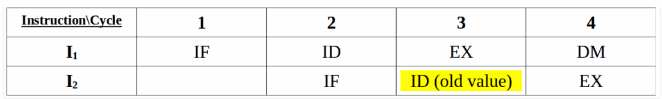To minimize data dependency stalls in the pipeline, operand forwarding is used.

Operand Forwarding : In operand forwarding, we use the interface registers present between the stages to hold intermediate output so that dependent instruction can access new value from the interface register directly.

Considering the same example:
I1 : ADD R1, R2, R3
I2 : SUB R4, R1, R2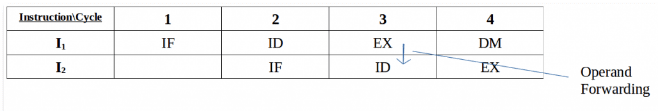Data Hazards

Data hazards occur when instructions that exhibit data dependence, modify data in different stages of a pipeline. Hazard cause delays in the pipeline. There are mainly three types of data hazards:

1) RAW (Read after Write) [Flow dependency]
2) WAR (Write after Read) [Anti-Data dependency]
3) WAW (Write after Write) [Output dependency]

Let there be two instructions I and J, such that J follow I. Then,

• RAW hazard occurs when instruction J tries to read data before instruction I writes it.
Eg:
I: R2 <- R1 + R3 J: R4 <- R2 + R3
• WAR hazard occurs when instruction J tries to write data before instruction I reads it.
Eg:
I: R2 <- R1 + R3 J: R3 <- R4 + R5
• WAW hazard occurs when instruction J tries to write output before instruction I writes it.
Eg:
I: R2 <- R1 + R3 J: R2 <- R4 + R5

WAR and WAW hazards occur during the out-of-order execution of the instructions.

## Pipelining | (Types and Stalling)

Types of pipeline

• Uniform delay pipeline
In this type of pipeline, all the stages will take same time to complete an operation.
In uniform delay pipeline, Cycle Time (Tp) = Stage Delay

If buffers are included between the stages then, Cycle Time (Tp) = Stage Delay + Buffer Delay

• Non-Uniform delay pipeline
In this type of pipeline, different stages take different time to complete an operation.
In this type of pipeline, Cycle Time (Tp) = Maximum(Stage Delay)

For example, if there are 4 stages with delays, 1 ns, 2 ns, 3 ns, and 4 ns, then

Tp = Maximum(1 ns, 2 ns, 3 ns, 4 ns) = 4 ns

If buffers are included between the stages,

Tp = Maximum(Stage delay + Buffer delay)

Example : Consider a 4 segment pipeline with stage delays (2 ns, 8 ns, 3 ns, 10 ns). Find the time taken to execute 100 tasks in the above pipeline.
Solution : As the above pipeline is a non-linear pipeline,
Tp = max(2, 8, 3, 10) = 10 ns
We know that ETpipeline = (k + n – 1) Tp = (4 + 100 – 1) 10 ns = 1030 ns

NOTE: MIPS = Million instructions per second

Performance of pipeline with stalls

Speed Up (S) = Performancepipeline / Performancenon-pipeline
=> S = Average Execution Timenon-pipeline / Average Execution Timepipeline
=> S = CPInon-pipeline * Cycle Timenon-pipeline / CPIpipeline * Cycle Timepipeline

Ideal CPI of the pipelined processor is ‘1’. But due to stalls, it becomes greater than ‘1’.
=> S = CPInon-pipeline * Cycle Timenon-pipeline / (1 + Number of stalls per Instruction) * Cycle Timepipeline

As Cycle Timenon-pipeline = Cycle Timepipeline,

Speed Up (S) = CPInon-pipeline / (1 + Number of stalls per instruction)

Offer running on EduRev: Apply code STAYHOME200 to get INR 200 off on our premium plan EduRev Infinity!

## GATE Computer Science Engineering(CSE) 2022 Mock Test Series

131 docs|167 tests

,

,

,

,

,

,

,

,

,

,

,

,

,

,

,

,

,

,

,

,

,

;